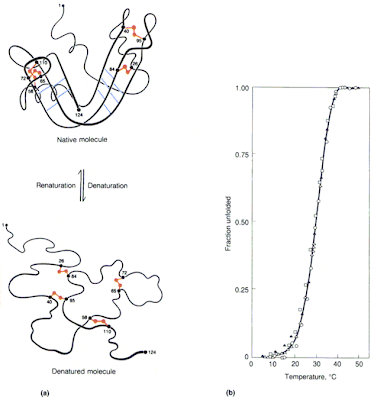## Sunday, September 6, 2009

### The power of thermodynamics

Chapter 6 of Nelson's Biological Physics ends with a nice extract from a 2001 Science paper describing optical tweezer experiments which reversibly unfold single RNA molecules.Figure b above shows how the amount of unfolded RNA as a function of applied force can be fit simply that predicted for a two-state equilibrium distribution (which has the same mathematical form as a Fermi-Dirac distribution!) The fit also gives a good estimate of the free energy of unfolding (about 80 k_B T at room temperature).

Nelson also gives simple arguments that give the probability distribution P(t) for the different waiting times t for folding and unfolding, P(t)=kexp(-kt) where k is the rate. The right panel of c. above shows the RNA length as a function of time for different applied forces. The resulting range of distribution times (note they are the order of seconds) are shown in panel d.

What is real new and cool here is observing all this for single molecules. Decades ago people found that the two-state model could describe the folding and unfolding of many biomolecules.Here are the slides for a lecture I have given previously on how this two-state equilibria can quantitatively describe the unzipping of DNA and unfolding of proteins. The right panel figure above is just one example of a measurement the amount of unfolded molecule as function of temperature. It can be fit to the same two-state distribution.

As Nelson note, it is amazing and impressive that such incredibly simple expressions based on simple thermodynamic considerations can describe such complex systems and processes.